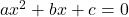# C++ Program to Solve Quadratic Equations

The C++ program to solve quadratic equations in standard form is a simple program based on the quadratic formula. Given the coefficients as input, it will solve the equation and output the roots of the equation.

This is a simple program is intended for intermediate level C++ programmers.

The program is compiled using Dev-C++ 4.9.9.2 version installed on a Windows 7 64-bit PC. You may try other standard C compilers and the program will still work if you use the correct C libraries.

## Problem Definition

In this program, we solve the quadratic equation using the formula.

\begin{aligned}
&x = \frac{-b \pm \sqrt{b^2 - 4ac}}{2a}\\\\
&When\\\\
&ax^2 + bx + c = 0
\end{aligned}

Where a, b and c are coefficient of the equationwhich is in the standard form.

The steps to compute the quadratic equation is given below.

Step1:

The program requests the input coefficient values a, b and c. When the user input the values, it will compute two terms t1 and t3 and an intermediate term t2.

t1 = -1 * b

Step2:

The function term2 () is called in step 2 and returned value of function is assigned to t2. The term2 () function receives the coefficient values – a, b, c and compute the value for t2.

t2 = term2(a, b, c);

The term () function returns and assign value of b<sup>2</sup> – 4ac to t2 and it is useful in understanding the root of the quadratic equation.

For example,

If (t2 < 0), then the roots are not real

If (t2 == 0) then, there is exactly one root value.

If (t2 > 0) then there are two root values.

The above condition is checked and printed with output values. Now we need to compute the roots and display the output values.

Step3:

A term t3 is assigned value after taking square root of t2.

t3 = sqrt (t2);

Step4:

Finally, we have t1 and t3 to compute two roots of a quadratic equation.

root1 = (t1 + t3)/ 2 * a;
root2 = (t1 + t3)/ 2 * a;

Then root1 and root are calculated and printed immediately.

## Flowchart – Program for Quadratic Equations

To understand flow of logic of this program, see the flowchart below.

## Program Code – Program for Quadratic Equation

/* C Program to solve a quadratic equation - Ax^2 + Bx + C = 0 using the formula
X1= -b + sqrt (b^2 – 4 * a * c)/ (2 * a)
and X2 = -b - sqrt (b^2 – 4 * a * c)/ (2* a) */

#include <stdio.h>
#include <stdlib.h>
#include <iostream.h>
#include <cmath.h>

using namespace std;
int main (){

int term2 (int a, int b, int c);

/* coefficients a, b, c */
int a, b, c;
float root1, root2, t1, t2, t3;
root1 = root2 = 0.0;
cout << "\n\n\n\n\n\n";
cout << "\t\t\t\tPlease enter coefficients:" << endl;
cout << "\t\t\t\tA="; cin >> a;
cout << "\t\t\t\tB="; cin >> b;
cout << "\t\t\t\tC="; cin >> c;
cout << "\t\t\t\tA=" << " " << a;
cout << "\tB=" << " " << b;
cout << "\tC=" << " " << c << endl;
cout << endl;

//Compute roots
t1 = -1 * b;
t2 = term2 (a, b, c);
t3 = sqrt (t2);
root1 = (t1 + t3)/ (2 * a);
root2 = (t1 - t3)/ (2 * a);

//Display roots
cout << "\t\t\t\tRoot-1 =" << " " << root1 << endl;
cout << "\t\t\t\tRoot-2 =" << " " << root2 << endl;
getch ();
return EXIT_SUCCESS;
}

int term2 (int x, int y, int z){
int p = y * y;
int q = 4 * x * z;
int r = p - q;
if (r < 0)
cout << "\t\t\t\tThere is no real root." << endl;
if (r == 0)
cout << "\t\t\t\tThere is only one real root." << endl;
if (r > 0)
cout << "\t\t\t\tThere are two real roots." << endl;
return r;
}

## Output

The output of the above program is given below.

                    Please enter coefficients:
A=1
B=22
C=44
A= 1      B= 22    C= 44

There are two real roots:
Root-1 = -2.22504
Root-2 = -19.775